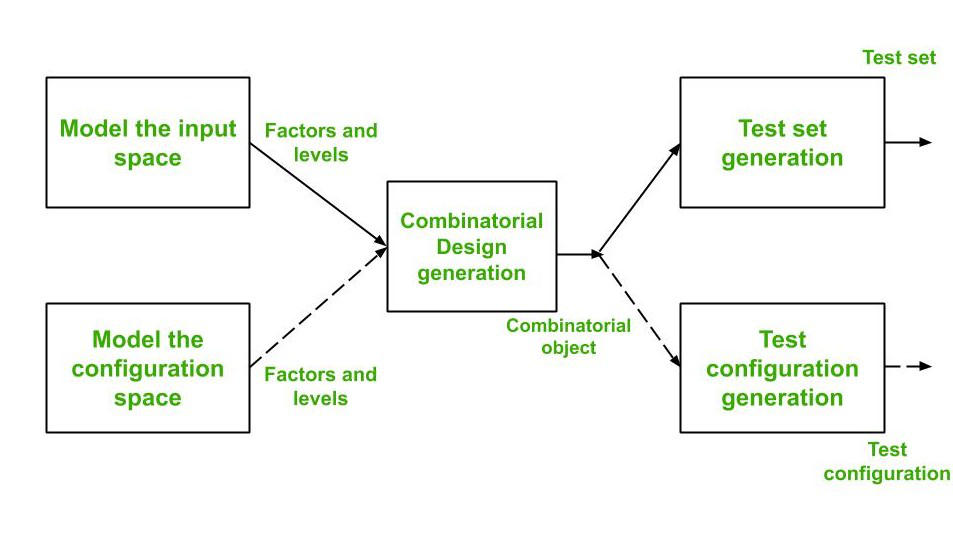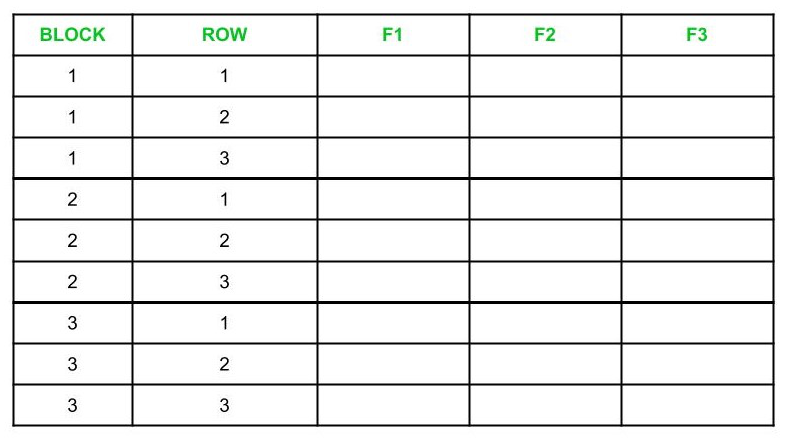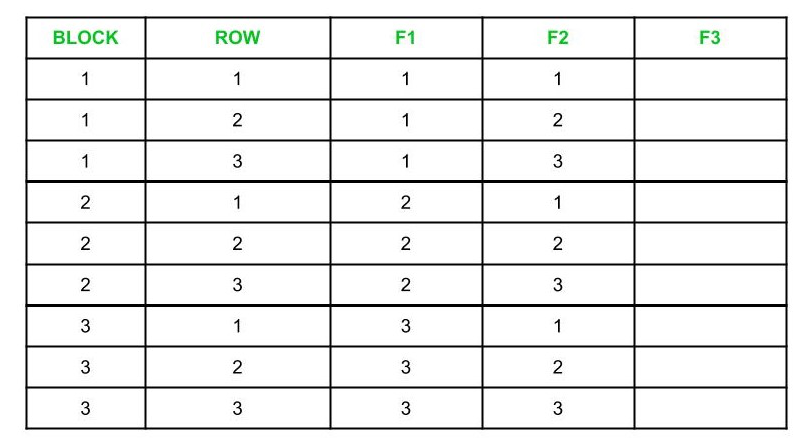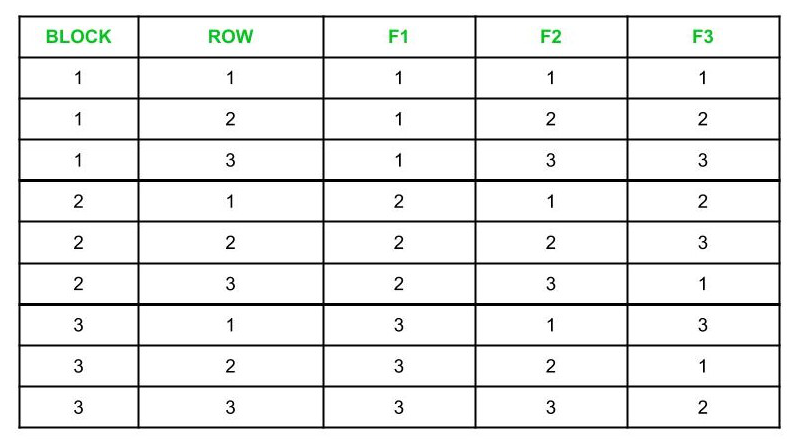# Test Configuration Generation in Combinatorial Testing

Test configuration generation is an important part of Combinatorial test design process. Combinatorial design process is shown in the figure below :As can be seen from the above figure, combinatorial test design process has 3 main steps :

• Modeling of the input space and configuration space.
• Generation of a combinatorial object with the help of a combinatorial design procedure. Combinatorial object obtained is an array of factors and levels.
• Generation of a test set or test configuration using the combinatorial object.

Note :
The input variables are called factors and values that are assignable to a factor is called a level.

Test Design Algorithm :
To design test configurations, we make use of a Test Design algorithm. This algorithm takes as input n number of factors (input variables) and outputs a set of factor combinations such that all level pairs are covered. Test Design algorithm has the following steps :

1. Relabel the Factors :
The given factors must first be relabelled as F1, F2, F3, ….. Fn such that :

```|F1| >= |F2| >= |F3| >= .........|Fn-1| >= |Fn|

Let b = |F1| and k = |F2|

Here, |Fi| is the number of levels in Factor Fi
```
2. Prepare a Table :
Create a table containing n number of columns labeled as F1, F2, F3, …..Fn, and (b x k) number of rows divided into b number of blocks. Here, each block contains k number of rows. A sample table is shown below for n = b = k = 3 :3. Fill the columns F1 and F2 :
We fill the column F1 with 1s in Block 1, 2s in Block 2, and similarly for other blocks. For column F2, we fill the block 1 with the sequence 1, 2, 3 …. k in rows 1 through k and repeat this for the rest of the blocks. A sample table is shown below :4. Find MOLS of order k and fill the rest of the columns :
Before filling the columns, we must first understand MOLS and how to find them.

MOLS (Mutually Orthogonal Latin Squares) are used for selecting a subset of factor combinations from a complete set. A Latin square of order n is an n x n matrix where no element appears more than once in a row and column.

Example-1 :
If S = {X, Y}, then Latin squares of order 2 will be :

```X  Y
Y  X

and

Y  X
X  Y
```

Example-2 :
If S = {1, 2, 3}, then Latin squares of order 3 will be :

```1  2  3
2  3  1
3  1  2

and

2  3  1
1  2  3
3  1  2

and

2  1  3
3  2  1
1  3  2
```

To construct a Latin square, create the first row with n distinct elements and fill the additional rows by permuting the first row. For example,

If S = {1, 2, 3, 4}, then a Latin square could be :

```1  2  3  4
2  3  4  1
3  4  1  2
4  1  2  3
```

To create MOLS, let M1 and M2 be two Latin squares, each of order n.

Let M1 ( i, j ) and M2 ( i, j ) denote element at the ith row and jth column in Latin squares M1 and M2 respectively. Now, we create an n x n matrix L from M1 and M2 such that L ( i, j ) is M1 ( i, j ) M2 ( i, j ), i.e. we juxtapose corresponding elements of M1 and M2.

If each element in L appears exactly once, i.e. it is unique, then M1 and M2 are said to be mutually orthogonal Latin squares of order n.

For example,

```                1  2  3                2  3  1
Consider M1 =   2  3  1    and, M2 =   1  2  3
3  1  2                3  1  2
```

So, L will be constructed as follows:

```        12  23  31
L =     21  32  13
33  11  22
```

Since elements in L are unique, so, M1 and M2 are MOLS of order 3.

Note :
When n is a prime number or a power of a prime number, then MOLS(n) contains n -1 MOLS.
Also, MOLS do not exist for n = 2 and n = 6, however, they do exist for all other values greater than 2.

Now, we can find MOLS of order k in order to fill remaining columns. Number these MOLS as M1, M2, and so on.
Here, s < k for k > 1, where s = number of MOLS of order k. Fill Block 1 of column F3 with elements from column 1 of M1, Block 2 with elements from column 2 of M1, and so on.

If b > k, then reuse columns of M1 to fill rows in remaining (b-k) blocks. This process is repeated for columns F4 through Fn using MOLS M2 through Ms. If s < n – 2, then we can fill remaining columns by randomly selecting values of the factors.

For example,

```If n = k = 3
```

Then MOLS of order 3 are :

```         1  2  3                 1  2  3
M1 =     2  3  1    and, M2 =    3  1  2
3  1  2                 2  3  1
```

We can fill remaining columns of the table using these MOLS. Refer to table shown below for a better understanding.5. Check if constraints are satisfied :
If there are no constraints given, then Step 5 and 6 should be skipped. Otherwise, entries in rows should be marked with a box if they do not satisfy constraints given. Constraints could be given like :

• Safari browser supports only Mac OS.
• Firefox browser works on Windows, Linux.
• Windows OS supports only LAN and PPP protocols.

6. Remove configurations that do not satisfy given constraints :
The configurations that are highlighted with a box in the table have to be removed. This is done by following a two-step procedure to remove them and retain pairwise coverage :

1. Modify the highlighted rows so that the constraints are maintained.
2. Add new configurations that cover the pairs that were left uncovered while replacing the highlighted rows.

7. Replace numbers in columns with given factor values :
In this step, we finally obtain test configurations by replacing values in columns of table with the actual values for factors. For example,

If F1 is a factor called Operating Systems and levels of F1 includes {MacOs, Windows, Linux}

Then if column F1 has values like:

```2
3
1 ```

Then, they should be replaced as follows :

```Windows
Linux
MacOS
```

where Windows, Linux and MacOS represent 2, 3 and 1 respectively.

After performing this step for all columns, the table obtained will contain the final test configurations.

Master Software Testing and Automation in an efficient and time-bound manner by mentors with real-time industry experience. Join our Software Automation Course and embark on an exciting journey, mastering the skill set with ease!
What We Offer:
• Comprehensive Software Automation program
• Expert Guidance for Efficient Learning
• Hands-on Experience with Real-world Projects
• Proven Track Record with 10,000+ Successful Geeks

Previous
Next